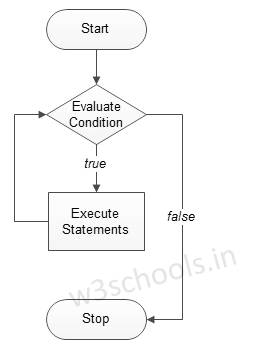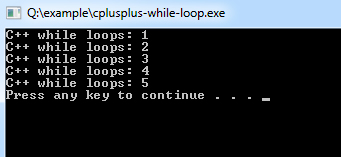# C++ while loops

C++ while loops statement allows to repeatedly run the same block of code until a condition is met.

while loop is a most basic loop in C++. while loop has one control condition, and executes as long the condition is true.  The condition of the loop is tested before the body of the loop is executed, hence it is called an entry-controlled loop.

The basic format of while loop statement is:

Syntax:
``````While (condition)
{
statement(s);
Incrementation;
}``````

Figure - Flowchart of while loop:### Example of a C++ Program to Demonstrate while loop

Example:
``````#include <iostream>
using namespace std;

int main ()
{
/* local variable Initialization */   int n = 1,times=5;

/* while loops execution */   while( n <= times )
{
cout << "C++ while loops: " << n <<endl;
n++;
}
return 0;
}``````
Program Output: